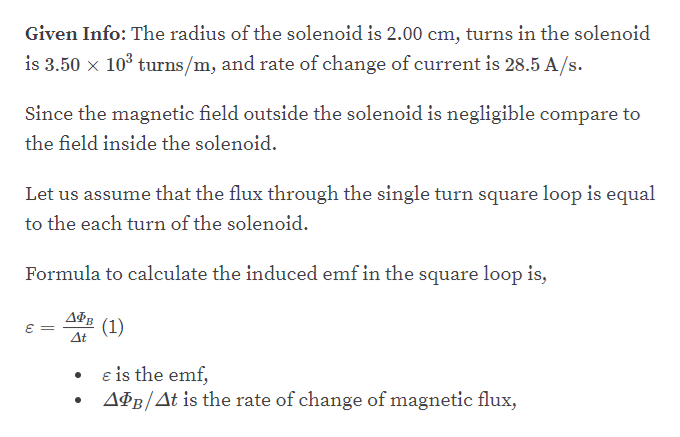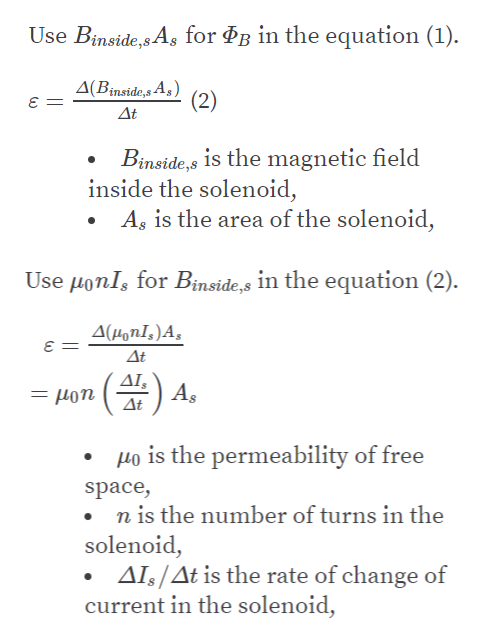Question
1 views

A long solenoid of radius r = 2.00 cm is wound with
3.50 x 103 turns/m and carries a current that changes at the
rate of 28.5 A/s as in Figure P20.60. What is the magnitude of
the emf induced in the square conducting loop surrounding
the center of the solenoid?

check_circle

Step 1help_outlineImage TranscriptioncloseGiven Info: The radius of the solenoid is 2.00 cm, turns in the solenoid is 3.50 x 10° turns/m, and rate of change of current is 28.5 A/s. Since the magnetic field outside the solenoid is negligible compare to the field inside the solenoid. Let us assume that the flux through the single turn square loop is equal to the each turn of the solenoid. Formula to calculate the induced emf in the square loop is, (1) At e is the emf, APB/At is the rate of change of magnetic flux, fullscreen
Step 2help_outlineImage TranscriptioncloseUse Binside,s As for &g in the equation (1). A(Binside,s A5) (2) At Binside,s is the magnetic field inside the solenoid, A, is the area of the solenoid, Use µonIs for Binside,s in the equation (2). A(HonI,)A, At (#) 4. ΔΙ Hon Δt Ho is the permeability of free space, • n is the number of turns in the solenoid, AIs/At is the rate of change of current in the solenoid, fullscreen
Step 3

### Want to see the full answer?

See Solution

#### Want to see this answer and more?

Solutions are written by subject experts who are available 24/7. Questions are typically answered within 1 hour.*

See Solution
*Response times may vary by subject and question.
Tagged in